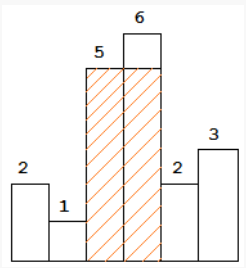Problem title
Difficulty
Avg time to solve

Ceil from BST
Easy
15 mins
Median of two sorted arrays
Hard
25 mins
Minimum Parentheses
Easy
10 mins
Covid Vaccination
Moderate
--
Minimum Elements
Moderate
40 mins
Target Sum
Moderate
--
Moderate
30 mins
Minimum Subarray With Required Sum
Moderate
18 mins
Return Subsets Sum to K
Moderate
40 mins
Delete Kth node From End
Moderate
15 mins23

# Largest rectangle in a histogram

Difficulty: HARD
Avg. time to solve
25 min
Success Rate
75%

Problem Statement

#### You are supposed to return the area of the largest rectangle possible in the given histogram.

##### For example :
``````In the below histogram where array/list elements are {2, 1, 5, 6, 2, 3}.
````````````The area of largest rectangle possible in the given histogram is 10.
``````
##### Input format :
``````The first line contains a single integer ‘T’ denoting the number of test cases.

The first line of each test case contains a single integer ‘N’ denoting the number of elements in the array/list.

The second line contains ‘N’ single space-separated integers denoting the elements of the array/list.
``````
##### Output format :
``````For each test case, print an integer denoting the area of the largest rectangle possible in the given histogram.
``````
##### Note :
``````You do not need to print anything; it has already been taken care of. Just implement the given function.
``````
##### Constraints :
``````1 <= T <= 10
1 <= N <= 10^6
0 <= HEIGHTS[i] <= 10^9

Where ‘T’ is the number of test cases.
'N' is the length of the given array/list.
And, HEIGHTS[i] denotes the height of the 'ith' histogram bar.

Time Limit: 1 sec.
``````
##### Sample Input 1 :
``````2
10
1 0 1 2 2 2 2 1 0 2
10
1 2 1 0 1 1 0 0 2 2
``````
##### Sample Output 1 :
``````8
4
``````
##### Explanation For Sample Input 1 :
``````In the first test case, the area of the largest rectangle of the given histogram is 8 in the rectangle starting from index 4 to index 7 in the given array/list.

In the second test case, the area of the largest rectangle of the given histogram is 4 in the rectangle starting from index 9 to index 10 in the given array/list.
``````
##### Sample Input 2 :
``````2
10
8 6 3 5 0 0 4 10 2 5
10
6 1 8 10 5 7 0 4 5 8
``````
##### Sample Output 2 :
``````12
20
``````
##### Explanation For Sample Input 2 :
``````In the first test case, the area of the largest rectangle of the given histogram is 12.

In the second test case, the area of the largest rectangle of the given histogram is 20.
``````Console